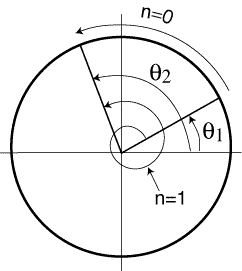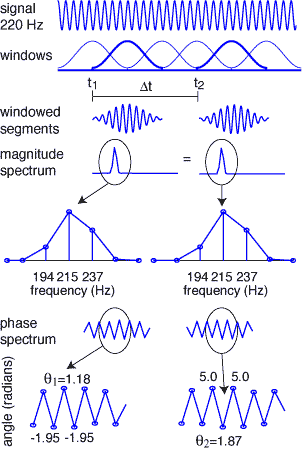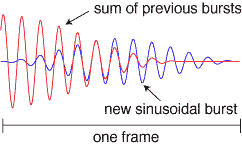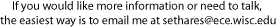A Phase Vocoder in Matlab### What is a Phase Vocoder?

The phase vocoder is a variation on the short-time Fourier transform.that uses phase information to improve the frequency estimates. It is ideal for use in applications such as time-stretching and/or time compression of audio, though there are a number of other special effects that can be implemented using the phase-vocoder strategy. This website describes the phase vocoder and presents an implementation in Matlab, along with a number of sound examples that demonstrate the operation. If you would like more detail (and better looking mathematical formulas) then you can download a chapter from my forthcoming book Rhythm and Transforms which describes the PV and the STFT (among other things).

 A short-time Fourier transform (STFT) signal processor is an analysis/synthesis method that begins by windowing a signal into short segments. The FFT is applied to each segment separately and the resulting spectral snapshot can be manipulated in a variety of ways. After the desired spectral changes, the resynthesis is handled by the inverse FFT to return each segment to the time domain.The modified segments are then summed. For the special case where no spectral manipulations are made (as shown), the output of the STFT is identical to the input. The frequency resolution of the FFT is: (1) resolution in Hz = (sampling rate)/(window size). In typical use, the support of the window (the region over which it is nonzero) is between 512 and 4096 samples. Using a medium window of size 2048 and a sampling rate of 44.1 KHz, the resolution in frequency is about 21.5 Hz. This may be adequate to specify high frequencies (where 21.5 Hz is a small percentage of the frequency in question) but it is far too coarse at the low end. A low note on the piano may have a fundamental near 80 Hz. The resolution of this FFT is only good to within 25%! For comparison, the distance between consecutive notes on the piano is a constant 6%. Is there a way to improve the frequency resolution of the STFT without overly harming the time resolution? Fortunately, the answer is "yes." The phase vocoder makes improved frequency estimates by using phase information that the STFT ignores.To see how the analysis portion of the PV can use phase information to make improved frequency estimates, suppose there is a sinusoid of unknown frequency but with known phases: at time t1 the sinusoid has phase theta1 and at time t2 it has phase theta2. The situation is depicted on the left. The sinusoid may have a frequency that moves it directly from theta1 to theta2 in time t2-t1. Or it may begin at theta1, move completely around the circle, and end at theta2 after one full revolution. Or it may revolve twice, or n times.In other words, the frequency multiplied by the change in time must equal the change in angle, that is, 2 pi f (t2-t1) = theta2 - theta1 or some 2 pi multiple. Solving for f gives (2) fn = (theta2 - theta1 + 2 pi n)/ (2 pi (t2-t1)) for some integer n. Without more information, it is not possible to uniquely determine f, though it is constrained to one of the above values.
 The phase vocoder exploits equation (2) by locating a common peak in the magnitude spectrum of two different frames. It then chooses the fn that is closest to the frequency of that peak. This is shown diagrammatically on the right where the signal is assumed to be a single sinusoid that spans the time interval over which the calculations are made. The output of the windowing is a collection of short sinusoidal bursts. The FFT is applied to each burst, resulting in magnitude and phase spectra. For the case of a pure sinusoidal input, the magnitude spectra of the successive spectra are the same (as shown).But the phase spectra differ, and these provide the needed values of theta1 (the phase corresponding to the peak of the first magnitude spectrum) and theta2 (the phase corresponding to the peak of the second magnitude spectrum). The time difference t2-t1 can be determined directly from the window length, the overlap factor, and the sampling rate. These values are then substituted into (2) and the fn that is closest in frequency to the peak is the PV's frequency estimate.To see the PV in action, and to give an idea of its accuracy, consider the problem of estimating the frequency of a 220 Hz sinusoid using a 2K FFT (assuming a sampling rate of 44.1 KHz and using an overlap of 2). According to equation (1), the resolution of the FFT is 21.5 Hz, that is, it is only possible to find the frequency of the sinusoid to within about 10 Hz. Indeed, the nearby frequencies that are exactly representable are 193.8, 215.3, and 236.9, as shown in the enlargement of the magnitude spectrum above. Since the peak at 215.3 is the largest, an actual error of 4.7 Hz occurs when using only the FFT magnitude. The PV improves this by exploiting phase information. The phases corresponding to the peaks at 215.3 are theta1=1.18 and theta2=1.87 and so

fn =(theta2 - theta1 + 2 pi n)/ (2 pi (t2-t1)) = ((1.18-1.87 + 2 pi n)/(2 pi 0.07)

With these values, the first six fn are 47.7472, 90.8136, 133.8800, 176.946, 220.0129, and 263.0793. Clearly, the fifth term is closest to 215.3 and the error in the frequency estimate is 0.0129, a vast improvement over 4.7 Hz. This kind of accuracy is typical and is not just a numerical fluke. In fact, Puckette and Brown  show that, under certain conditions (for a signal consisting of a single sinusoid and with a t2-t1 corresponding to a single sample) the phase vocoder estimate of the frequency is closely related to the maximum likelihood estimate.

In more complex situations, when the input signal consists of many sine waves, the phase manipulations are repeated for each peak individually, which is justified as long as the peaks are adequately separated in frequency. Once the analysis portion is complete, it is possible to change the signal in a variety of ways: by modifying the rate at which time passes (spacing the output bursts differently from the input bursts), by changing the frequencies in the signal (so that the output will contain different frequencies than the input), by adding or by subtracting partials.

### Resynthesis Using the Phase Vocoder

Once the modifications are complete, it is necessary to synthesize the output waveform. One possibility is to use a straightforward additive-synthesis where the partials (each with its desired frequency and amplitude) are generated individually and then summed together. This is computationally intensive when there are a large number of partials. Fortunately, there is a better way: the PV creates a complex-valued (frequency) vector. This can be inverted using the IFFT and the resulting output bursts are time-shifted and summed as in the STFT.

 Specification of the magnitude spectrum of the output is straightforward since it can be inherited directly from the input. The phase values are chosen to ensure continuity of the most prominent partials through successive bursts, as shown on the right for a single sinusoid. For each peak j in the magnitude spectrum, the required phase can be calculated directly from the frequency fj and the time interval between frame k and frame k-1. This is theta_k^j = theta_k-1^j + 2 pi fj (t_k - t_{k-1}). It is also necessary to choose the nearby phases (those under the same peak in the magnitude spectrum). If these are chosen to be theta_k^j + mod(n,2) pi (where n is the number of bins away from the peak value), the burst generated by the IFFT will be windowed with tapered ends, as on the right.For example, in the phase spectrum plots above, the values to the left and right of theta1 and theta2 are (approximately) either pi or 0 away.You can find my version of a phase vocoder here. You can also download a chapter from from my forthcoming book Rhythm and Transforms that contains more detail about the operation of the STFT and the PV.